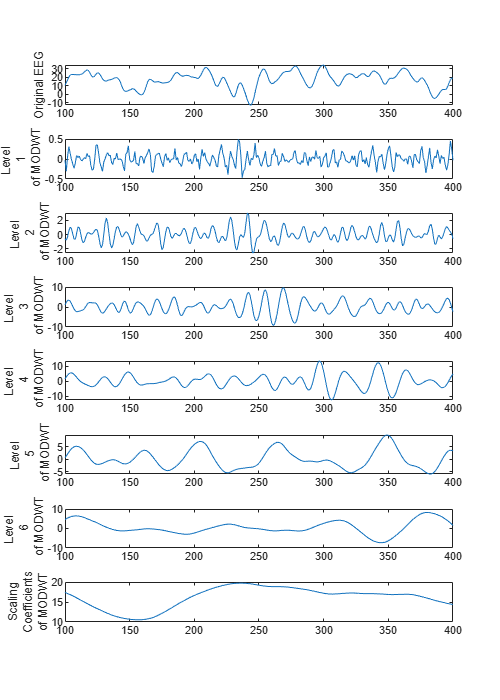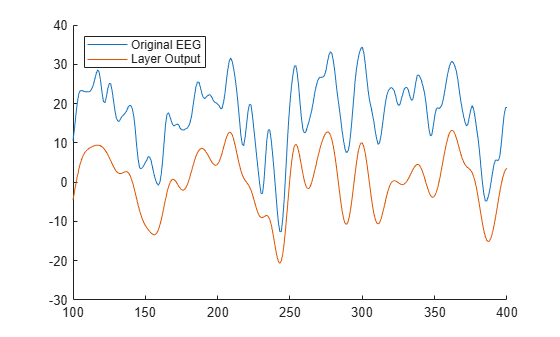# modwtLayer

Maximal overlap discrete wavelet transform (MODWT) layer

Since R2022b

## Description

A MODWT layer computes the MODWT and MODWT multiresolution analysis (MRA) of the input. Use of this layer requires Deep Learning Toolbox™.

## Creation

### Syntax

``layer = modwtLayer``
``layer = modwtLayer(Name=Value)``

### Description

````layer = modwtLayer` creates a MODWT layer. By default, the layer computes the MODWTMRA to level 5 using the Daubechies least-asymmetric wavelet with four vanishing moments (`'sym4'`) .The input to `modwtLayer` must be a `dlarray` (Deep Learning Toolbox) object in `"CBT"` format. The size of the time dimension of the tensor input must be greater than or equal to 2Level, where Level is the transform level of the MODWT. `modwtLayer` formats the output as `"SCBT"`. For more information, see Layer Output Format. NoteThe object initializes the weights internally for use as wavelet filters in the MODWT. It is not recommended to initialize the weights directly. ```

example

````layer = modwtLayer(Name=Value)` creates a MODWT layer with properties specified by name-value arguments. For example, ```layer = modwtLayer(Wavelet="haar")``` creates a MODWT layer that uses the Haar wavelet. You can specify the wavelet and the level of decomposition, among others.```

## Properties

expand all

### MODWT

Name of an orthogonal wavelet used in the MODWT, specified as a character vector or a string scalar.

Orthogonal wavelets are designated as type 1 wavelets in the wavelet manager. Valid built-in orthogonal wavelet families begin with `'haar'`, `'dbN'`, `'fkN'`, `'coifN'`, `'blN'`, `'hanSR.LP'`, `'symN'`, `'vaid'`, or `'beyl'`. Use `waveinfo` with the wavelet family short name to see supported values for any numeric suffixes and how to interpret those values. For example, `waveinfo("han")`.

For a wavelet specified by wname, the associated lowpass and highpass filters `Lo` and `Hi`, respectively, are `[~,~,Lo,Hi] = wfilters(wname)`.

Data Types: `char` | `string`

Initial wavelet filter pair, specified as a pair of even-length real-valued vectors. The lengths of the vectors must be equal. `LowpassFilter` and `HighpassFilter` must correspond to the lowpass and highpass filters, respectively, associated with an orthogonal wavelet. You can use `isorthwfb` to determine orthogonality.

```[~,~,Lo,Hi] = wfilters("db2"); [tf,checks] = isorthwfb(Lo,Hi);```
If unspecified, both filters default to `[]` and `modwtLayer` uses `Wavelet` to determine the filters used in MODWT.

You cannot specify both a wavelet name and a wavelet filter pair.

Example: ```layer = modwtLayer('LowpassFilter',Lo,'HighpassFilter',Hi)```

Data Types: `single` | `double`

Transform level to compute the MODWT, specified as a positive integer less than or equal to `floor(log2(N))`, where N is the size of the layer input in the time dimension.

Data Types: `single` | `double`

Boundary condition to use in the computation of the MODWT, specified as one of these:

• `"periodic"` — The signal is extended periodically along the time dimension. The number of wavelet coefficients equals the size of the signal in the time dimension.

• `"reflection"` — The signal is reflected symmetrically along the time dimension at the terminal end before computing the MODWT. The number of wavelet coefficients returned is twice the length of the input signal.

Selected levels for `modwtLayer` to output, specified as a vector of positive integers less than or equal to `Level`.

Data Types: `single` | `double`

Include lowpass coefficients, specified as a numeric or logical `1` (`true`) or `0` (`false`). If specified as `true`, the MODWT layer includes the `Level`th level lowpass (scaling) coefficients in the MODWT, or `Level`th level smooth in the MODWTMRA.

Data Types: `logical`

Aggregate selected levels, specified as a numeric or logical `1` (`true`) or `0` (`false`). If specified as `true`, the MODWT layer aggregates the selected levels and lowpass level (if `IncludeLowpass` is `true`) of each input channel by summation. If `AggregateLevels` is `true`, the size of the output in the spatial dimension is `1`. For more information, Compare modwtLayer Output with modwt and modwtmra Outputs.

Data Types: `logical`

Algorithm `modwtLayer` uses to compute the output, specified as one of these:

• `"MODWTMRA"` — Compute the maximal overlap discrete wavelet transform based multiresolution analysis.

• `"MODWT"` — Compute the wavelet coefficients of the maximal overlap discrete wavelet transform.

### Layer

Multiplier for weight learning rate, specified as a nonnegative scalar. If not specified, this property defaults to zero, resulting in weights that do not update with training. You can also set this property using the `setLearnRateFactor` (Deep Learning Toolbox) function.

The learnable parameter `'Weights'` in `modwtLayer` is a two-row matrix of the current filter pair. The first row is the lowpass filter and the second row is the highpass filter. By default, the weights are the lowpass and highpass filters associated with the default wavelet and do not update.

Data Types: `single` | `double`

Layer name, specified as a character vector or a string scalar. For `Layer` array input, the `trainnet` (Deep Learning Toolbox), `trainNetwork` (Deep Learning Toolbox), `assembleNetwork` (Deep Learning Toolbox), `layerGraph` (Deep Learning Toolbox), and `dlnetwork` (Deep Learning Toolbox) functions automatically assign names to layers with the name `""`.

The `modwtLayer` object stores this property as a character vector.

Data Types: `char` | `string`

Number of inputs to the layer, returned as `1`. This layer accepts a single input only.

Data Types: `double`

Input names, returned as `{'in'}`. This layer accepts a single input only.

Data Types: `cell`

Number of outputs from the layer, returned as `1`. This layer has a single output only.

Data Types: `double`

Output names, returned as `{'out'}`. This layer has a single output only.

Data Types: `cell`

## Examples

collapse all

Create a MODWT layer to compute the multiresolution analysis for the input signal. Use a coiflet wavelet with order 5. Set the transform level to 8. Only keep the details at levels 3, 5, and 7, and the approximation.

```layer = modwtLayer(Wavelet="coif5",Level=8, ... SelectedLevels=[3,5,7],Name="MODWT");```

Create a `dlnetwork` object containing a sequence input layer, a MODWT layer, and an LSTM layer. For a level-8 decomposition, set the minimum sequence length to 2^8 samples. To work with an LSTM layer, a flatten layer is also needed before the LSTM layer to collapse the spatial dimension into the channel dimension.

```mLength=2^8; sqLayer = sequenceInputLayer(1,Name="input",MinLength=mLength); layers = [sqLayer layer flattenLayer lstmLayer(10,Name="LSTM") ]; dlnet = dlnetwork(layers);```

Run a batch of 10 random single-channel signals through the `dlnetwork` object. Inspect the size and dimensions of the output. The flatten layer has collapsed the spatial dimension.

```dataout = dlnet.forward(dlarray(randn(1,10,2000,'single'),'CBT')); size(dataout)```
```ans = 1×3 10 10 2000 ```
`dims(dataout)`
```ans = 'CBT' ```

Load the Espiga3 electroencephalogram (EEG) dataset. The data consists of 23 channels of EEG sampled at 200 Hz. There are 995 samples in each channel. Save the multisignal as a `dlarray`, specifying the dimensions in order. `dlarray` permutes the array dimensions to the `"CBT"` shape expected by a deep learning network.

```load Espiga3 [N,nch] = size(Espiga3); x = dlarray(Espiga3,"TCB");```

Use `modwt` and `modwtmra` to obtain the MODWT and MRA of the multisignal down to level 6. By default, `modwt` and `modwtmra` use the `sym4` wavelet.

```lev = 6; wt = modwt(Espiga3,lev); mra = modwtmra(wt);```

Compare with `modwt`

Create a MODWT layer that can be used with the data. Set the transform level to 6. Specify the layer to use MODWT to compute the output. By default, the layer uses the `sym4` wavelet.

`mlayer = modwtLayer(Level=lev,Algorithm="MODWT");`

Create a two-layer `dlnetwork` object containing a sequence input layer and the MODWT layer you just created. Treat each channel as a feature. For a level-6 decomposition, set the minimum sequence length to 2^6.

```mLength = mlayer.Level; sqInput = sequenceInputLayer(nch,MinLength=2^mLength); layers = [sqInput mlayer]; dlnet = dlnetwork(layers);```

Run the EEG data through the `forward` method of the network.

`dataout = forward(dlnet,x);`

The `modwt` and `modwtmra` functions return the MODWT and MRA of a multichannel signal as a 3-D array. The first, second, and third dimensions of the array correspond to the wavelet decomposition level, signal length, and channel, respectively. Convert the network output to a numeric array. Permute the dimensions of the network output to match the function output. Compare the network output with the `modwt` output.

```q = extractdata(dataout); q = permute(q,[1 4 2 3]); max(abs(q(:)-wt(:)))```
```ans = 8.4402e-05 ```

Choose a MODWT result from `modwtLayer`. Compare with the corresponding channel in the EEG data. Plot each level of the `modwtLayer` output. Different levels contain information about the signal in different frequency ranges. The levels are not time aligned with the original signal because the layer uses the MODWT algorithm.

```channel = 10; t = 100:400; subplot(lev+2,1,1) plot(t,Espiga3(t,channel)) ylabel("Original EEG") for k=2:lev+1 subplot(lev+2,1,k) plot(t,q(k-1,t,channel)) ylabel(["Level ",k-1," of MODWT"]) end subplot(lev+2,1,lev+2) plot(t,q(lev+1,t,channel)) ylabel(["Scaling","Coefficients","of MODWT"]) set(gcf,Position=[0 0 500 700])```Compare with `modwtmra`

Create a second network similar to the first network, except this time specify that `modwtLayer` use the MODWTMRA algorithm and aggregate the fourth, fifth, and sixth levels. Do not include the lowpass level in the aggregation.

```sLevels = [4 5 6]; mlayer = modwtLayer(Level=lev, ... SelectedLevels=sLevels, ... IncludeLowpass=0, ... AggregateLevels=1); layers = [sqInput mlayer]; dlnet2 = dlnetwork(layers);```

Run the EEG data through the `forward` method of the network. Convert the network output to a numeric array. Permute the dimensions as done previously.

```dataout = forward(dlnet2,x); q = extractdata(dataout); q = permute(q,[1 4 3 2]);```

Aggregate the fourth, fifth, and sixth levels of the MRA. Compare with the network output.

```mraAggregate = sum(mra(sLevels,:,:)); max(abs(q(:)-mraAggregate(:)))```
```ans = 2.1036e-04 ```

Inspect a MODWTMRA result from the layer. Compare with the corresponding channel in the EEG data. By choosing only the fourth, fifth, and six levels, and not including the lowpass component, the layer removes several high and low frequency components from the signal. The transformed signal is smoother than the original signal and the low frequency components are removed so that the offset is closer to 0. The output is time aligned with the original signal because the layer uses the default MODWTMRA algorithm. Depending on your goal, preserving time alignment can be useful.

```channel = 10; t = 100:400; figure hold on plot(t, Espiga3(t,channel)) plot(t,q(1,t,1,channel)) hold off legend(["Original EEG", "Layer Output"], ... Location="northwest")```expand all

## Version History

Introduced in R2022b